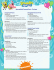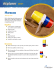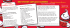# Rolling Cups - the Mathematics Assessment Project

## Transcription

Rolling Cups - the Mathematics Assessment Project
```PROBLEM SOLVING
Mathematics Assessment Project
CLASSROOM CHALLENGES
A Formative Assessment Lesson
Modeling Motion:
Rolling Cups
Mathematics Assessment Resource Service
University of Nottingham & UC Berkeley
For more details, visit: http://map.mathshell.org
© 2015 MARS, Shell Center, University of Nottingham
May be reproduced, unmodified, for non-commercial purposes under the Creative Commons license
detailed at http://creativecommons.org/licenses/by-nc-nd/3.0/ - all other rights reserved
Modeling Motion: Rolling Cups
MATHEMATICAL GOALS
This lesson unit is intended to help you assess how well students are able to:
• Choose appropriate mathematics to solve a non-routine problem.
• Generate useful data by systematically controlling variables.
• Develop experimental and analytical models of a physical situation.
COMMON CORE STATE STANDARDS
This lesson relates to all the Standards for Mathematical Practice in the Common Core State
Standards for Mathematics, with a particular emphasis on Practices 1, 2, 3, 4, 5, 7, and 8:
1. Make sense of problems and persevere in solving them.
2. Reason abstractly and quantitatively.
3. Construct viable arguments and critique the reasoning of others.
4. Model with mathematics.
5. Use appropriate tools strategically.
6. Attend to precision.
7. Look for and make use of structure.
8. Look for and express regularity in repeated reasoning.
This lesson gives students the opportunity to apply their knowledge of the following Standards for
Mathematical Content in the Common Core State Standards for Mathematics:
F-BF: Build a function that models a relationship between two quantities.
G-MG: Apply geometric concepts in modeling situations.
G-GMD: Visualize relationships between two-dimensional and three-dimensional objects.
G-SRT: Prove theorems involving similarity.
A-CED: Create equations that describe numbers or relationships.
INTRODUCTION
•
•
•
Before the lesson, students watch a video of Rolling Cups. They then work on a related task
designed to assess their current approaches to modeling. You collect and review students’ work
and write questions to help improve their models.
During the lesson, students first work individually to review their initial work. They then work in
pairs to compare their approaches to the task, choosing a strategy that will produce an improved
model and together implement that strategy. Students next review some sample work before
discussing as a class the strengths and weaknesses of different approaches to the modeling task.
In a follow-up lesson, students review their work on the task.
MATERIALS REQUIRED
•
•
•
•
•
Each student will need a copy of the task sheet: Modeling Rolling Cups.
Each pair of students will need a fresh copy of the task sheet, Modeling Rolling Cups, copies of
each of the Sample Responses to Discuss, a large sheet of paper, and a felt-tipped pen.
It is desirable that each pair of students has access to a computer with the applet Modeling with
Geometry: Rolling Cups. If this is not possible the lesson can still be conducted with one class
computer and a data projector.
Supply paper and plastic cups, rules, scrap paper, graph paper, protractors, string, chalk, glue,
scissors, and calculators for students who choose to use them.
For the whole-class discussions, you will need a computer with data projector and two
plastic/paper cups that have different base diameters and heights.
TIME NEEDED
30 minutes before the lesson, a 90-minute lesson (or two 50-minute lessons), and 20 minutes in a
follow-up lesson. All timings are approximate and will depend on the needs of your students.
Teacher guide
Modeling Motion: Rolling Cups
T-1
BEFORE THE LESSON
Introduction to Modeling Rolling Cups (15 minutes)
We suggest you take time to explore carefully some different solution strategies to this problem
before teaching the lesson. Introduce the problem scenario to students. We describe two ways of
doing this:
(i) Practical demonstration
Show students a cup and make sure they understand the terms ‘slant height’, ‘perpendicular height’,
‘narrow diameter’, and ‘wide diameter’.
Show the students a paper cup and indicate the slant side of the cup.
I’m going to lay this cup on its slant side and push it. What do you think will happen?
Roll the cup.
Tell me what you see.
the top of the cup (widest diameter) rolls.
Do you think the circle this cup rolls will have a bigger or smaller roll radius? Why?
Check students’ predictions by rolling the cup.
(ii) Using the computer data projector
Introduce the task using the video extracts on the MAP website:
http://map.mathshell.org/lesson_support/rolling_cups/
Display the introductory slide, The Task. Ask students to make some predictions:
Which of these objects do you think will roll the largest circle? Why?
What about the second largest circle?
What will happen when the soup can is rolled?
Accept all student answers without comment.
Show the four videos: Short Glass, Plastic cup, Tall Glass, Soup can.
As I play each video, try to guess what will happen!
Were you surprised by any of the results?
Note: At this stage, do not show the Cup Rolling Calculator. That is for the main lesson.
Teacher guide
Modeling Motion: Rolling Cups
T-2
Individual work on the assessment task: Modeling Rolling Cups (15 minutes)
Give each student a copy of the Modeling
Modeling Rolling Cups
Rolling Cups
Student Materials
+,-(
I would like you to think about how to solve
this problem. Think about what you’ve seen
so far.
Explain what you want students to do.
I would like you to spend 15 minutes
Make sure you explain your work clearly.
At the top of the sheet is the data you saw
in the four videos, together with some more
data.
,(-*&'(")*+*#
!"#\$%&"'%&("%("%)*\$&(
."/\$(
3022'4(
/"0#\$1\$2( /"0#\$1\$2(
Beta version March 2012
560%1(
6\$%71*(
8'66(
20/",&(
9(
:!(
:(
:"(
;<#(
=(
:(
;(
:!(
>?!(
+(
;!(
;(
@"(
;A"(
!(
:(
:(
B#(
C%D"%"1\$E(
F(
:(
;(
<(
>A(
G(
:!(
;(
:"(
A"(
H(
:"(
:(
:"(
>A"(
I(
:!(
?(
:"(
:"(
./"0+&1*(23+&
!"##\$%&
'(")*+*#
Here is a reminder of the data you saw in the video with a few extra cups added.
1. Describe how each of the three lengths on the picture affect the roll radius.
Show how you used the data to explain your ideas.
2. Show how you can use math to predict the radius of the circle rolled by any size of cup.
Show all your reasoning, including any diagrams and calculations.
Slide P-1 of the projector resource may be projected as you make these points.
It is important that, as far as possible, students are allowed to answer the question without assistance.
If students are struggling to get started, help them understand what is required, but make sure you do
not do the task for them. The first few questions on the Common issues table, on the next page, may
be useful.
Students who sit together often produce similar answers, so that, when they come to compare their
work, they have little to discuss. For this reason we suggest that, when students do the task
individually, you ask them to move to different seats. At the beginning of the formative assessment
lesson allow them to return to their usual seats. Experience has shown that this produces more
profitable discussions because students have varied approaches to discuss.
© 2012 MARS University of Nottingham UK
S-1
When all students have made a reasonable attempt at the task, tell them that they will have time to
revisit and revise their solutions later.
Do not be too concerned if you found this work difficult, as we will have a lesson tomorrow that
Assessing students’ responses
Collect students’ responses to the task. Make some notes on what their work reveals about their
current modeling strategies.
We suggest that you do not score students’ work. Research shows that this will be counterproductive,
as it will encourage students to compare scores and distract their attention from what they can do to
improve their mathematics. Instead, help students make further progress by using a list of questions
and prompts that summarize ways to develop their modeling strategies. Some suggestions for these
are given in the Common issues table on page T-4. We suggest you make a list of your own questions,
based on your students’ work. We recommend you either:
•
write one or two questions on each student’s work, or
give each student a printed version of your list of questions and highlight the questions for each
individual student.
If you do not have time to do this, you could select a few questions that will be of help to the majority
of students and write these on the board when you return the work to the students at the beginning of
the lesson.
•
Teacher guide
Modeling Motion: Rolling Cups
T-3
Common issues:
Suggested questions and prompts:
Has some idea of the effect of the variables but has not
considered how they interact (Q1)
For example: The student says that if the wide diameter is
made bigger, then the cup rolls in a smaller circle.
Does not control the variables (Q1)
For example: The student draws a conclusion by
comparing two cups with no dimensions in common.
Makes no use of the data (Q1&2)
For example: The student makes no reference to the data
in their discussion of the problem.
Does not consider all the variables (Q1&2)
For example: The student only considers slant height in
their hypothesis about which cup rolls the larger circle.
Or: The student omits reference to one variable.
Has no modeling strategy (Q2)
For example: The student has written/drawn little.
Does not move on from data production
For example: The student organizes the data to vary
quantities systematically but does not analyze for patterns.
Or: The student notices a pattern but does not try to record
it algebraically.
Requests further information
For example: The student says they cannot solve the
problem because there is insufficient information.
Only draws a diagram
For example: The student makes a scale drawing without
explanation.
• Is this true whatever the narrow diameter value?
• What happens if the narrow diameter is equal to
the wide diameter?
• What do you think would happen if you fixed
the narrow diameter and slant height and varied
the wide diameter?
• Could you reorganize the data from the video to
• Which measures occur in the data from the
video?
• Are there any other measures that might be
useful?
• What do you already know? What are you
trying to find?
• Could you draw a diagram? Where is the roll
related to the measures in the problem?
pattern?
• Can you see any patterns? Describe the pattern
you see in words.
• How could you record what happens in the
pattern every time using algebra?
• What are the extreme cases? How would they
show in a formula?
• What other data do you want? Describe the
experiments you want to do. How will that
• How will you use your diagram/scale drawing to
solve the problem?
Or: The student sketches the cup, identifying key
variables.
Provides a model
For example: The student uses scale drawings to predict
which cup will roll the larger circle.
Or: The student writes a formula.
Teacher guide
• You predict that … How could you check this?
• Does your model make a correct prediction for
each of the cups in the table?
• Would your model work for any cup? How do
you know?
Modeling Motion: Rolling Cups
T-4
SUGGESTED LESSON OUTLINE
Introduction and individual work (10 minutes)
Remind students of their work on Modeling Rolling Cups.
Your work today will be to produce a mathematical solution to Modeling Rolling Cups.
There are lots of different ways of doing this!
Give the students their scripts from the assessment task. If you have not written your questions on
individual scripts, display them on the board for the class to read.
your work. Spend a few minutes answering my questions. Then decide what you will do next to
solve this problem.
decision about a possible plan of action.
Introducing the Cup Rolling Calculator (5 minutes)
Show students the Cup Rolling Calculator using a data projector:
To improve your models, you could try rolling some cups. We also have a calculator that will
Demonstrate how to use the calculator:
What values could I choose for the narrow diameter? Where do I put that?
Which number or numbers do you want me to change?
What is the dependent variable?
Teacher note
The students’ next job is to work together to produce an improved solution to the task. There are two
ways to work on this: using a practical method, and/or using the computer calculator. The practical
method has the advantage that students can more readily visualize the 3-dimensional aspects of the
situation, which should help them move towards an analytical method. The computer calculator
generates data rapidly and helps students to find patterns and make generalizations.
Both approaches have their difficulties. Students may find it difficult to record on paper how the cup
rolls and to make accurate measurements. Some students may, therefore, opt to work with real cups,
string, chalk, and the sidewalk! The computer approach may lead to students getting buried in data
and will not help them develop explanations.
Teacher guide
Modeling Motion: Rolling Cups
T-5
It is worthwhile for students to try both approaches where possible. Everyone should have the
experience of rolling one cup and gathering data from it, if possible. The computer can then be used
Collaborative small-group work: modeling (30 minutes)
Ask students to form pairs and give each pair a fresh copy of the Modeling Rolling Cups task, a paper
cup, a rule, a protractor, a pencil, a large sheet of paper, and a felt-tipped pen.
You’re going to work in pairs today.
To begin, take turns to explain your work to the other student in your pair. When listening to your
partner’s solution, ask questions so you understand.
When your pair has shared solutions, choose a strategy to work on together. Take about 5
minutes to come up with a plan of work.
Work together to produce a joint solution to the problem that is better than either of your
individual solutions.
I have given you a paper cup and some equipment that you can use to help you visualize the
problem and collect some data on one cup. There is other equipment if you need it. There is also
the Rolling Cup Calculator that you can use to collect additional data.
Plan your data collection before you start, so that you don’t collect too much!
These instructions are summarized on Slide P-2 of the projector resource.
If there is only one computer and data projector, explain that when students have discussed their ideas
in their pairs for a while, they should write a list of any additional data they want collecting. You will
collect this data for them using the computer.
During the collaborative small-group work, you have two tasks: to notice students’ approaches to the
task and to support their modeling activity.
Notice students’ approaches to the task
What kind of models do the students attempt to make? Do they produce an empirical model based on
data? Do they analyze the data for patterns and then record those algebraically to produce a formula?
Or do they produce an analytical model using geometry?
When using the computer calculator, do students control the variables, keeping some fixed and
varying others systematically? Do students recognize the need to go beyond data production? Do they
consider extreme cases such as ‘wide diameter = narrow diameter’ or ‘narrow diameter = 0’? Do they
choose useful representations, such as tables or graphs? Do they identify patterns in the data and try
to record these algebraically? Do they try to identify a general rule or formula from their data?
When using a practical approach by rolling a cup, do students record the path of a cup on a surface
using e.g. chalk? Do they attempt to draw scale diagrams? Do they produce an analytical geometric
model using similar triangles? Do they distinguish ‘slant height’ and ‘perpendicular height’? Do they
recognize what role height plays in their geometrical models?
Support students’ modeling activity
There are many ways to tackle this task. Try not to prompt students towards using a particular
approach. Instead, ask questions that help students develop and clarify their thinking while following
their chosen modeling strategy.
It is important to recognize that after producing data, some students may struggle to identify how to
move forward. They may need to be reassured that this struggle is just part of the modeling process.
Teacher guide
Modeling Motion: Rolling Cups
T-6
Students themselves need to figure out the math they know that is relevant to this situation, and
formulate a strategy for moving forward. By engaging with that struggle, students can experience
being real problem solvers, rather than just solving problems with information and strategies given to
them.
Why did you choose these values for the narrow/wide diameter? What other data might be
useful?
What happens if… you make the narrow diameter much bigger than/close in size to/smaller than
the wide diameter? … you increase the wide diameter but keep the narrow diameter fixed?
Can you see any patterns in the data? Use your patterns to predict new results. Check to see if
you are correct.
How would you write that pattern using algebra? What does that tell you about a formula for the
How else could you represent your data to help understand the relationships? [Graphs, tables,
diagrams, scale drawings.]
How do you think the roll radius depends on the narrow diameter / the wide diameter / the slant
length?
Try to combine the patterns you have learned about into a formula to help you predict the size of
Extending the lesson over two days
If you are taking two days to complete the lesson unit then you may want to end the first lesson here.
At the start of the second day, allow students time to familiarize themselves with their joint solution
before moving on to the collaborative analysis of sample responses.
Collaborative analysis of Sample Responses to Discuss (30 minutes)
The sample responses will help students to think further about their own approaches and confront
them with approaches they may have not yet considered.
Give each group of students a copy of all three Sample Responses to Discuss.
Choose one of the sample solutions. Read it carefully and then work together to answer the
questions.
When you have finished your work on one sample solution, choose another.
It is important that students work in depth through a couple of the Sample Responses to Discuss, to
see different strengths in contrasting approaches. This is more important than covering all of the
material.
Whilst students are analyzing the Sample Responses to Discuss, support their thinking as before.
What approach is Gerry taking?
How might Heather use that data to complete a formula?
How does Judi’s diagram connect with Gerry’s?
The three modeling strategies shown in the Sample Responses to Discuss are described in detail on
the next page. You may want to use some of this information to support the whole-class discussion at
the end of the lesson.
Teacher guide
Modeling Motion: Rolling Cups
T-7
Heather uses the computer calculator to
generate data. She generates the data by
fixing two variables, W and N, and varying
S to see the impact on R. She analyses
some of her data to find patterns and uses
the patterns to define aspects of the
formula for R. She interprets some of the
formula and data in the context of the
problem. The way Heather sets out her
data on the page is important. She aligns
values so that it is possible to see how
systematic changes in N and W result in
patterned change in R. These are strengths
in her solution.
Heather might usefully continue with her systematic exploration of values of N and W by looking at
N=2, W=3, N=3, W=4, and so on. She might then be able to see patterns in the resulting values of R.
Graphing values of S, R for sets of values of N with fixed W, or W with fixed N might be useful
However, Heather could complete the formula using her data on N=0.
She claims that R =
S ×?
.
W −N
She knows that when N=0, the cup is a cone and it does not matter what W is. In that case, the
formula must make R =
€
S ×?
SW
SW
. The formula is thus R =
.
= S gives R =
W −0
W −0
W −N
Some of Heather’s argument is strong: she links the real cups she has seen with empirical data,
patterns in the data, and the formula. However, her presentation is a bit disorganized and she does not
bring all the
€ pieces of her argument
€ together.
€
The disorganized nature of Heather’s work may be a useful stimulus for discussion with students on
the differences between the notes they produce in working towards a solution and the reorganization
of materials required to present and
communicate a solution to others.
Gerry uses a simple, accessible strategy
that gives a partial solution to the
problem. Given any cup, Gerry could
draw a scale diagram that would allow
him to make an approximate measure of
the roll radius, depending on the accuracy
of the scale drawing. However, Gerry’s
approach must be used each time; it is not
a general solution.
Gerry provides a little explanation of how
to move from a cup to a cone, and from a
cone to a triangle. He does not explain
how he knows that his triangle is
isosceles.
Teacher guide
Modeling Motion: Rolling Cups
T-8
Asking students to use Gerry’s method enables them to engage with his construction problem: how,
given these measures, do you construct an isosceles triangle with an embedded isosceles trapezium.
The value of R found given the values of N, W, and S should be about 4½".
The advantage of Gerry’s method is that it is easy to understand. Some difficulties with Gerry’s
method are that the construction method needs to be used accurately to produce a reliable measure for
the roll radius and the method does not produce a general solution: a scale drawing must be produced
for each cup. As a result, his solution is not easily reversible, e.g. it would be difficult constructing the
triangle, knowing the roll radius to find specific values of N, W and S.
Judi draws a diagram showing the
cup in two dimensions as a pair of
similar triangles. She says that the
cup scenario can be modeled this
way, but gives no explanation of
how to move from a cup to the
diagram.
Throughout, Judi uses capital letters
to represent variables. While not
incorrect, this may be confusing as
the labels for vertices are also
capitals. It is more conventional to
use lower case for variables.
Judi claims that Triangle ACE is
similar to Triangle BCD but does not explain how she knows this. Overall, although she has chosen
quite sophisticated mathematics to use, she provides almost no explanation of her work.
The argument for similar triangles could be made as follows:
Angle BCD is common to both triangles.
Slicing the cone perpendicular to the ground, the face is a triangle. The wide diameter and narrow
diameter included in that triangle are parallel because they are in the same plane (slice) and
because the top of the cup is (assumed) to be parallel to the base. So W is parallel to N.
Then Angle EAB = Angle DBC.
Since the sum of the angles in a triangle is always 180º, the third angles must also be congruent.
So as the three angles are equal, the triangles must be similar.
Since the triangles are similar, corresponding side lengths are in the same ratio.
Hence
R R−S
=
W
N
Judi makes a standard error in the manipulation of this formula:
€
€
€
RN = WR − S
Judi’s working can be corrected and her method used to find a formula for R.
RN = W ( R − S ) and RN = WR −WS .
Teacher guide
€
Modeling Motion: Rolling Cups
T-9
Thus R(W − N ) = SW and R =
€
SW
.
(W − N )
A full solution would include making sense of the formula in the context, by, for example, showing
what happens for values of W and N that produce the extreme cases of the cone and cylinder.
€
distinguish squashing a cup flat from slicing the cup perpendicular to the
ground!
Whole-class discussion: comparing different approaches (15 minutes)
Ask students to compare the work they have seen on this problem and to identify the different
strengths of the various methods. Slides P-3, P-4, and P-5 of the projector resource contain the three
Sample Responses to Discuss and can be used to support this discussion.
Describe one strategy for solving the problem. Did any of you solve the problem in that way?
What are the strengths of that method? Were there any difficulties with that method?
Describe another strategy for solving the problem.
Which method did you prefer? Why?
Ask students to review their work on the modeling problem across the lesson:
What was your initial strategy for solving this problem?
Has your strategy changed during the lesson? How? Why?
What advice would you give to someone just starting on this problem?
Follow-up lesson: individual review (20 minutes)
Give each student a copy of the How Did You Work? questionnaire. This is intended to provoke
students to reflect on and make comparisons between the methods used in this lesson.
carefully as you can.
Some teachers give this as a homework task.
Teacher guide
Modeling Motion: Rolling Cups
T-10
SOLUTIONS
Rolling Cups
1. This question is designed to see whether students
can make deductions from the data by controlling
variables.
They can deduce that:
If the wide diameter is increased and other variables
held constant, then the roll radius will decrease.
This may be deduced from the data for cups A and G.
If the narrow diameter is increased (and other
variables held constant), then the roll radius will
increase.
This may be deduced from the data for cups A and F.
Student Materials
Beta version March 2012
Modeling Rolling Cups
+,-(
9(
,(-*&'(")*+*#
!"#\$%&"'%&("%("%)*\$&(
."/\$(
3022'4(
/"0#\$1\$2( /"0#\$1\$2(
560%1(
6\$%71*(
8'66(
20/",&(
;<#(
:!(
:(
:"(
=(
:(
;(
:!(
+(
;!(
;(
@"(
;A"(
!(
:(
:(
B#(
C%D"%"1\$E(
F(
:(
;(
<(
>A(
>?!(
G(
:!(
;(
:"(
A"(
H(
:"(
:(
:"(
>A"(
I(
:!(
?(
:"(
:"(
./"0+&1*(23+&
!"##\$%&
'(")*+*#
Here is a reminder of the data you saw in the video with a few extra cups added.
1. Describe how each of the three lengths on the picture affect the roll radius.
Show how you used the data to explain your ideas.
2. Show how you can use math to predict the radius of the circle rolled by any size of cup.
Show all your reasoning, including any diagrams and calculations.
If the slant height is increased (and other variables held constant), then the roll radius will increase.
This may be deduced from the data for cups B and E.
If the narrow diameter is zero, the roll radius is equal to the slant height of the cup. This can be
deduced from cup H.
2. This question is best answered using a geometrical construction. Your students may, however,
devise other, equally valuable approaches.
© 2012 MARS University of Nottingham UK
The diagram shows a cup ABCD on its side. It rolls in a
A
The wide diameter = w
The narrow diameter = n
The slant height = s
B
w
n
Triangles AOD and BOC are similar, since <DAO =
<CBO (AD is parallel to BC) and <AOD is common.
Therefore:
S-1
D
C
s
O
r
r r−s
=
w
n
⇔ rn = rw − sw
⇔ sw = r(w − n)
⇔r=
sw
w−n
This result shows that that the roll radius is proportional to the slant height. It also shows that when n
and w are nearly equal then the roll radius becomes very large, as is shown by the tin can!
Teacher guide
Modeling Motion: Rolling Cups
T-11
Modeling Rolling Cups
Here is a reminder of the data you saw in the video with a few extra cups added.
1. Describe how each of the three lengths on the picture affect the roll radius.
Show how you used the data to explain your ideas.
2. Show how you can use math to predict the radius of the circle rolled by any size of cup.
Show all your reasoning, including any diagrams and calculations.
Student materials
Modeling Motion: Rolling Cups
© 2015 MARS, Shell Center, University of Nottingham
S-1
Sample Responses to Discuss: Heather
Student materials
Modeling Motion: Rolling Cups
© 2015 MARS, Shell Center, University of Nottingham
S-2
What values for the narrow diameter and wide diameter do you think Heather should choose next?
Heather has described some patterns in the data.
Find and describe a new pattern in the data.
What are the strengths of Heather’s solution?
What would you do to improve Heather’s solution?
Student materials
Modeling Motion: Rolling Cups
© 2015 MARS, Shell Center, University of Nottingham
S-3
Sample Responses to Discuss: Gerry
Student materials
Modeling Motion: Rolling Cups
© 2015 MARS, Shell Center, University of Nottingham
S-4
Use Gerry’s method to find the roll radius of this cup:
Wide diameter = 3"
Narrow diameter = 1"
Slant length = 3"
Gerry says he can use his method to find the roll radius of any cup.
Is this correct?
What are the strengths of Gerry’s approach?
What could Gerry do to improve his solution?
Student materials
Modeling Motion: Rolling Cups
© 2015 MARS, Shell Center, University of Nottingham
S-5
Sample Responses to Discuss: Judi
Student materials
Modeling Motion: Rolling Cups
© 2015 MARS, Shell Center, University of Nottingham
S-6
Judi says Triangle ACE and Triangle BCD are similar.
Is Judi correct?
Judi draws a triangle diagram.
Explain how this represents a cup.
Judi has made a mistake in her formula.
Find and correct the mistake.
Now find a formula for R, the roll radius.
Student materials
Modeling Motion: Rolling Cups
© 2015 MARS, Shell Center, University of Nottingham
S-7
How Did You Work?
1.
My own method was similar to one of the sample responses
OR
My own method was different from all of the sample responses
Because:
Because:
2.
Our group’s method was similar to sample response:
OR
Our group’s method was different from all of the sample responses
Because:
Because:
3. Explain giving reasons, whether or not you think the method(s) you used was more efficient/clearer than the sample response methods.
4. Explain how the method used by Heather is different to the method used by Gerry.
5. Write down the advantages and disadvantages of each of the methods shown in the sample responses.
Student materials
Modeling Motion: Rolling Cups
© 2015 MARS, Shell Center, University of Nottingham
S-8
Modeling Rolling Cups
Rolling Cups
Student Materials
Beta version March 2012
Modeling Rolling Cups
+,-(
,(-*&'(")*+*#
!"#\$%&"'%&("%("%)*\$&(
."/\$(
3022'4(
/"0#\$1\$2( /"0#\$1\$2(
560%1(
6\$%71*(
8'66(
20/",&(
9(
:!(
:(
:"(
;<#(
=(
:(
;(
:!(
>?!(
+(
;!(
;(
@"(
;A"(
!(
:(
:(
B#(
C%D"%"1\$E(
F(
:(
;(
<(
>A(
G(
:!(
;(
:"(
A"(
H(
:"(
:(
:"(
>A"(
I(
:!(
?(
:"(
:"(
./"0+&1*(23+&
!"##\$%&
'(")*+*#
Here is a reminder of the data you saw in the video with a few extra cups added.
Projector Resources
Modeling Motion: Rolling Cups
1. Describe how each of the three lengths on the picture affect the roll radius.
Show how you used the data to explain your ideas.
P-1
Collaborative small-group work
1.  Take turns to explain your work.
Ask questions if you don’t understand.
2.  Agree on a strategy for producing a joint
solution that is better than your individual
responses.
3.  Work together on implementing your strategy.
want to collect.
Projector Resources
Modeling Motion: Rolling Cups
P-2
Sample Responses to Discuss: Heather
Projector Resources
Modeling Motion: Rolling Cups
P-3
Sample Responses to Discuss: Gerry
Projector Resources
Modeling Motion: Rolling Cups
P-4
Sample Responses to Discuss: Judi
Projector Resources
Modeling Motion: Rolling Cups
P-5
Mathematics Assessment Project
Classroom Challenges
These materials were designed and developed by the
Shell Center Team at the Center for Research in Mathematical Education
University of Nottingham, England:
Malcolm Swan,
Nichola Clarke, Clare Dawson, Sheila Evans, Colin Foster, and Marie Joubert
with
Hugh Burkhardt, Rita Crust, Andy Noyes, and Daniel Pead
We are grateful to the many teachers and students, in the UK and the US,
who took part in the classroom trials that played a critical role in developing these materials
The classroom observation teams in the US were led by
David Foster, Mary Bouck, and Diane Schaefer
This project was conceived and directed for
The Mathematics Assessment Resource Service (MARS) by
Alan Schoenfeld at the University of California, Berkeley, and
Hugh Burkhardt, Daniel Pead, and Malcolm Swan at the University of Nottingham
Thanks also to Mat Crosier, Anne Floyde, Michael Galan, Judith Mills, Nick Orchard, and Alvaro
Villanueva who contributed to the design and production of these materials
This development would not have been possible without the support of
Bill & Melinda Gates Foundation
We are particularly grateful to
Carina Wong, Melissa Chabran, and Jamie McKee
The full collection of Mathematics Assessment Project materials is available from
http://map.mathshell.org
© 2015 MARS, Shell Center, University of Nottingham
This material may be reproduced and distributed, without modification, for non-commercial purposes,
All other rights reserved.
```

### Incycle cups microGREEN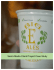### What you`ll need. How to put it all together.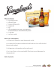### Onion Crackers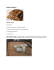### UK Barcelona FC Flyer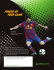### Kitchari Recipe - Kate Towell | Ayurveda and Holistic Wellness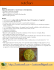### DIY Anemometer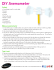### SpongeBob SquarePants - Recipes